# Piyush mahatma sem.2 PPT.pptx

31. May 2023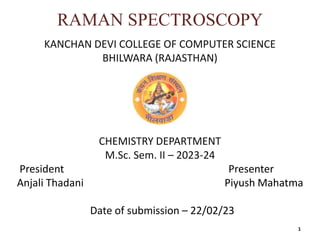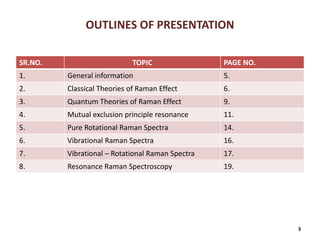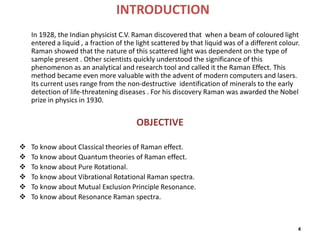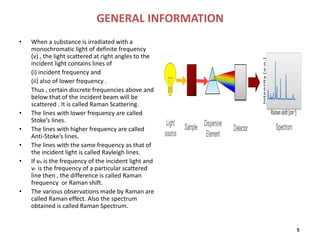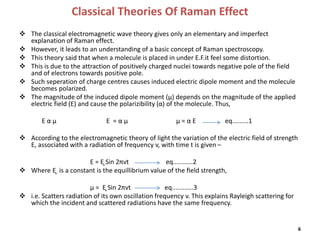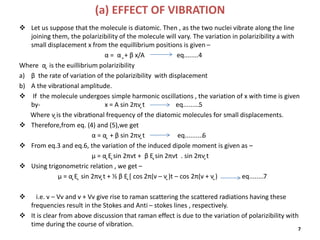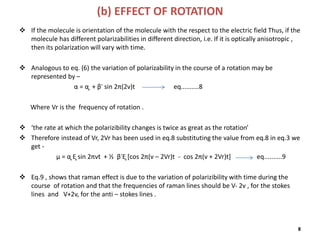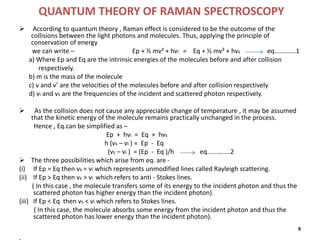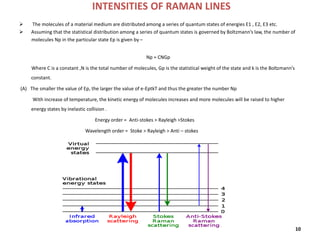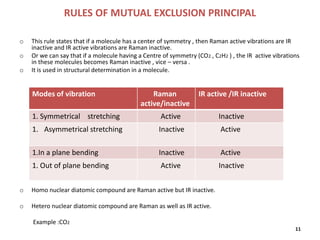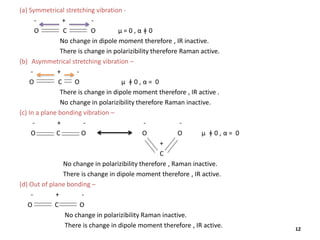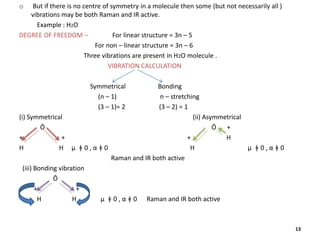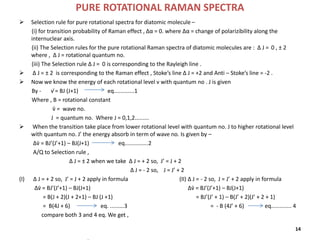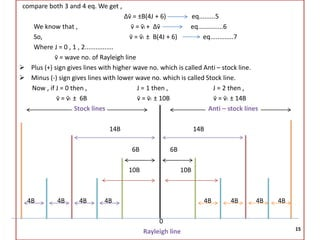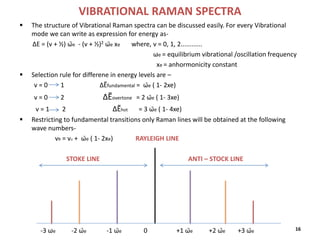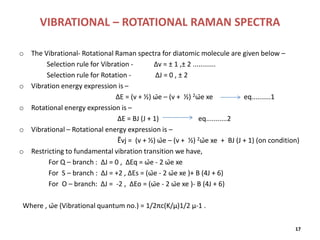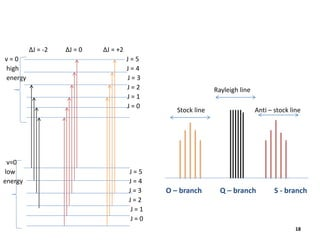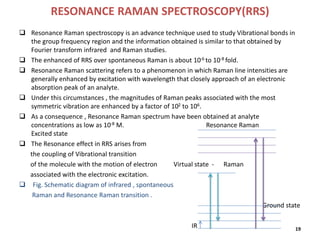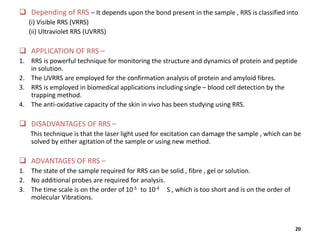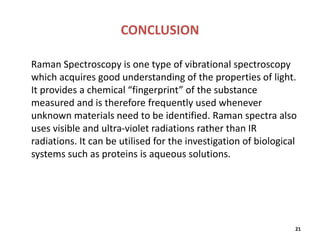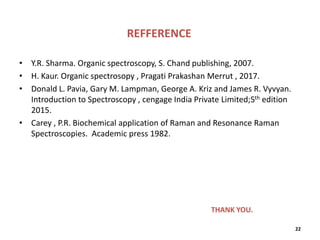1 von 22

### Piyush mahatma sem.2 PPT.pptx

• 1. RAMAN SPECTROSCOPY KANCHAN DEVI COLLEGE OF COMPUTER SCIENCE BHILWARA (RAJASTHAN) CHEMISTRY DEPARTMENT M.Sc. Sem. II – 2023-24 President Presenter Anjali Thadani Piyush Mahatma Date of submission – 22/02/23 1
• 2. Acknowledgment In the accomplishment of this project successfully, many people have best owned upon me their blessings and the heart pledge support , this time I am utilizing to thank all the people who have been concerned with project. Primarily I would thank god for being to complete this project with success. Then I would like to thank college Directors Shikha Bhadada and NaveenBhadada and H.O.D. of Science department Anupama Sharma, whose valuable guidance has been the ones that helped me patch this project and make it full proof success his suggestionsand his instructions has served as the major contributor towards the completion of the project. Then I would like to thank my parents and friends who have helped me with their valuable suggestionsand guidance has been helpful in various phases of the completion of the project. Last but not the least I would like to thank my classmates who have helped me a lot. 2
• 3. OUTLINES OF PRESENTATION SR.NO. TOPIC PAGE NO. 1. General information 5. 2. Classical Theories of Raman Effect 6. 3. Quantum Theories of Raman Effect 9. 4. Mutual exclusion principle resonance 11. 5. Pure Rotational Raman Spectra 14. 6. Vibrational Raman Spectra 16. 7. Vibrational – Rotational Raman Spectra 17. 8. Resonance Raman Spectroscopy 19. 3
• 4. INTRODUCTION In 1928, the Indian physicist C.V. Raman discovered that when a beam of coloured light entered a liquid , a fraction of the light scattered by that liquid was of a different colour. Raman showed that the nature of this scattered light was dependent on the type of sample present . Other scientists quickly understood the significance of this phenomenon as an analytical and research tool and called it the Raman Effect. This method became even more valuable with the advent of modern computers and lasers. Its current uses range from the non-destructive identification of minerals to the early detection of life-threatening diseases . For his discovery Raman was awarded the Nobel prize in physics in 1930. OBJECTIVE  To know about Classical theories of Raman effect.  To know about Quantum theories of Raman effect.  To know about Pure Rotational.  To know about Vibrational Rotational Raman spectra.  To know about Mutual Exclusion Principle Resonance.  To know about Resonance Raman spectra. 4
• 5. GENERAL INFORMATION • When a substance is irradiated with a monochromatic light of definite frequency (v) , the light scattered at right angles to the incident light contains lines of (i) incident frequency and (ii) also of lower frequency . Thus , certain discrete frequencies above and below that of the incident beam will be scattered . It is called Raman Scattering. • The lines with lower frequency are called Stoke’s lines. • The lines with higher frequency are called Anti-Stoke’s lines. • The lines with the same frequency as that of the incident light is called Rayleigh lines. • If vo is the frequency of the incident light and vr is the frequency of a particular scattered line then , the difference is called Raman frequency or Raman shift. • The various observations made by Raman are called Raman effect. Also the spectrum obtained is called Raman Spectrum. 5
• 6. Classical Theories Of Raman Effect  The classical electromagnetic wave theory gives only an elementary and imperfect explanation of Raman effect.  However, it leads to an understanding of a basic concept of Raman spectroscopy.  This theory said that when a molecule is placed in under E.F.it feel some distortion.  This is due to the attraction of positively charged nuclei towards negative pole of the field and of electrons towards positive pole.  Such seperation of charge centres causes induced electric dipole moment and the molecule becomes polarized.  The magnitude of the induced dipole moment (µ) depends on the magnitude of the applied electric field (E) and cause the polarizibility (α) of the molecule. Thus, E α µ E = α µ µ = α E eq.........1  According to the electromagnetic theory of light the variation of the electric field of strength E, associated with a radiation of frequency v, with time t is given – E = E ̥Sin 2πνt eq...........2  Where E ̥ is a constant is the equillibrium value of the field strength, µ = E ̥Sin 2πνt eq............3  i.e. Scatters radiation of its own oscillation frequency v. This explains Rayleigh scattering for which the incident and scattered radiations have the same frequency. 6
• 7. (a) EFFECT OF VIBRATION  Let us suppose that the molecule is diatomic. Then , as the two nuclei vibrate along the line joining them, the polarizibility of the molecule will vary. The variation in polarizibility a with small displacement x from the equillibrium positions is given – α = α ̥+ β x/A eq........4 Where α ̥ is the euillibrium polarizibility a) β the rate of variation of the polarizibility with displacement b) A the vibrational amplitude.  If the molecule undergoes simple harmonic oscillations , the variation of x with time is given by- x = A sin 2πν ̥t eq.........5 Where ν ̥is the vibrational frequency of the diatomic molecules for small displacements.  Therefore,from eq. (4) and (5),we get α = α ̥ + β sin 2πν ̥t eq..........6  From eq.3 and eq.6, the variation of the induced dipole moment is given as – µ = α ̥E ̥sin 2πνt + β E ̥sin 2πνt . sin 2πν ̥t  Using trigonometric relation , we get – µ = α ̥E ̥ sin 2πν ̥t + ½ β E ̥[ cos 2π(ν – ν ̥)t – cos 2π(ν + ν ̥) eq........7  i.e. v – Vv and v + Vv give rise to raman scattering the scattered radiations having these frequencies result in the Stokes and Anti – stokes lines , respectively.  It is clear from above discussion that raman effect is due to the variation of polarizibility with time during the course of vibration. 7
• 8. (b) EFFECT OF ROTATION  If the molecule is orientation of the molecule with the respect to the electric field Thus, if the molecule has different polarizabilities in different direction, i.e. If it is optically anisotropic , then its polarization will vary with time.  Analogous to eq. (6) the variation of polarizability in the course of a rotation may be represented by – α = α ̥ + β̕ sin 2π(2ν)t eq..........8 Where Vr is the frequency of rotation .  ‘the rate at which the polarizibility changes is twice as great as the rotation’  Therefore instead of Vr, 2Vr has been used in eq.8 substituting the value from eq.8 in eq.3 we get - µ = α ̥E ̥sin 2πνt + ½ β̕E ̥[cos 2π(ν – 2Vr)t - cos 2π(ν + 2Vr)t] eq..........9  Eq.9 , shows that raman effect is due to the variation of polarizibility with time during the course of rotation and that the frequencies of raman lines should be V- 2v , for the stokes lines and V+2v, for the anti – stokes lines . 8
• 9. QUANTUM THEORY OF RAMAN SPECTROSCOPY  According to quantum theory , Raman effect is considered to be the outcome of the collisions between the light photons and molecules. Thus, applying the principle of conservation of energy we can write – Ep + ½ mv² + hvi = Eq + ½ mv² + hvs eq............1 a) Where Ep and Eq are the intrinsic energies of the molecules before and after collision respectively. b) m is the mass of the molecule c) v and v’ are the velocities of the molecules before and after collision respectively d) vi and vs are the frequencies of the incident and scattered photon respectively.  As the collision does not cause any appreciable change of temperature , it may be assumed that the kinetic energy of the molecule remains practically unchanged in the process. Hence , Eq.can be simplified as – Ep + hvi = Eq + hvs h (vs – vi ) = Ep - Eq (vs – vi ) = (Ep - Eq )/h eq.............2  The three possibilities which arise from eq. are - (i) If Ep = Eq then vs = vi which represents unmodified lines called Rayleigh scattering. (ii) If Ep > Eq then vs > vi which refers to anti - Stokes lines. ( In this case , the molecule transfers some of its energy to the incident photon and thus the scattered photon has higher energy than the incident photon). (iii) If Ep < Eq then vs < vi which refers to Stokes lines. ( In this case, the molecule absorbs some energy from the incident photon and thus the scattered photon has lower energy than the incident photon). 9
• 10. INTENSITIES OF RAMAN LINES  The molecules of a material medium are distributed among a series of quantum states of energies E1 , E2, E3 etc.  Assuming that the statistical distribution among a series of quantum states is governed by Boltzmann’s law, the number of molecules Np in the particular state Ep is given by – Np = CNGp Where C is a constant ,N is the total number of molecules, Gp is the statistical weight of the state and k is the Boltzmann’s constant. (A) The smaller the value of Ep, the larger the value of e-EptkT and thus the greater the number Np With increase of temperature, the kinetic energy of molecules increases and more molecules will be raised to higher energy states by inelastic collision . Energy order = Anti-stokes > Rayleigh >Stokes Wavelength order = Stoke > Rayleigh > Anti – stokes 10
• 11. RULES OF MUTUAL EXCLUSION PRINCIPAL o This rule states that if a molecule has a center of symmetry , then Raman active vibrations are IR inactive and IR active vibrations are Raman inactive. o Or we can say that if a molecule having a Centre of symmetry (CO2 , C2H2 ) , the IR active vibrations in these molecules becomes Raman inactive , vice – versa . o It is used in structural determination in a molecule. o Homo nuclear diatomic compound are Raman active but IR inactive. o Hetero nuclear diatomic compound are Raman as well as IR active. Example :CO2 11 Modes of vibration Raman active/inactive IR active /IR inactive 1. Symmetrical stretching Active Inactive 1. Asymmetrical stretching Inactive Active 1.In a plane bending Inactive Active 1. Out of plane bending Active Inactive
• 12. (a) Symmetrical stretching vibration - - + - O C O µ = 0 , α ǂ 0 No change in dipole moment therefore , IR inactive. There is change in polarizibility therefore Raman active. (b) Asymmetrical stretching vibration – - + - O C O µ ǂ 0 , α = 0 There is change in dipole moment therefore , IR active . No change in polarizibility therefore Raman inactive. (c) In a plane bonding vibration – - + - - - O C O O O µ ǂ 0 , α = 0 + C No change in polarizibility therefore , Raman inactive. There is change in dipole moment therefore , IR active. (d) Out of plane bonding – - + - O C O No change in polarizibility Raman inactive. There is change in dipole moment therefore , IR active. 12
• 13. o But if there is no centre of symmetry in a molecule then some (but not necessarily all ) vibrations may be both Raman and IR active. Example : H2O DEGREE OF FREEDOM – For linear structure = 3n – 5 For non – linear structure = 3n – 6 Three vibrations are present in H2O molecule . VIBRATION CALCULATION Symmetrical Bonding (n – 1) n – stretching (3 – 1)= 2 (3 – 2) = 1 (i) Symmetrical (ii) Asymmetrical Ō Ō + + + + H H H µ ǂ 0 , α ǂ 0 H µ ǂ 0 , α ǂ 0 Raman and IR both active (iii) Bonding vibration Ō + + H H µ ǂ 0 , α ǂ 0 Raman and IR both active 13
• 14. PURE ROTATIONAL RAMAN SPECTRA  Selection rule for pure rotational spectra for diatomic molecule – (i) for transition probability of Raman effect , Δα = 0. where Δα = change of polarizibility along the internuclear axis. (ii) The Selection rules for the pure rotational Raman spectra of diatomic molecules are : Δ J = 0 , ± 2 where , Δ J = rotational quantum no. (iii) The Selection rule Δ J = 0 is corresponding to the Rayleigh line .  Δ J = ± 2 is corresponding to the Raman effect , Stoke’s line Δ J = +2 and Anti – Stoke’s line = -2 .  Now we know the energy of each rotational level ν with quantum no . J is given By - ν ̄= BJ (J+1) eq.............1 Where , B = rotational constant ν̄ = wave no. J = quantum no. Where J = 0,1,2.........  When the transition take place from lower rotational level with quantum no. J to higher rotational level with quantum no. J’ the energy absorb in term of wave no. Is given by – Δv̄ = BJ’(J’+1) – BJ(J+1) eq...............2 A/Q to Selection rule , Δ J = ± 2 when we take Δ J = + 2 so, J’ = J + 2 Δ J = - 2 so, J = J’ + 2 (I) Δ J = + 2 so, J’ = J + 2 apply in formula (II) Δ J = - 2 so, J = J’ + 2 apply in formula Δv̄ = BJ’(J’+1) – BJ(J+1) Δv̄ = BJ’(J’+1) – BJ(J+1) = B(J + 2)(J + 2+1) – BJ (J +1) = BJ’(J’ + 1) – B(J’ + 2)(J’ + 2 + 1) = B(4J + 6) eq. .........3 = - B (4J’ + 6) eq............. 4 compare both 3 and 4 eq. We get , 14
• 15. compare both 3 and 4 eq. We get , Δv̄ = ±B(4J + 6) eq.........5 We know that , v̄ = v̄i + Δv̄ eq..............6 So, v̄ = v̄i ± B(4J + 6) eq.............7 Where J = 0 , 1 , 2................ v̄ = wave no. of Rayleigh line  Plus (+) sign gives lines with higher wave no. which is called Anti – stock line.  Minus (-) sign gives lines with lower wave no. which is called Stock line. Now , if J = 0 then , J = 1 then , J = 2 then , v̄ = v̄i ± 6B v̄ = v̄i ± 10B v̄ = v̄i ± 14B Stock lines Anti – stock lines 14B 14B 6B 6B 10B 10B 4B 4B 4B 4B 4B 4B 4B 4B 0 Rayleigh line 15
• 16. VIBRATIONAL RAMAN SPECTRA  The structure of Vibrational Raman spectra can be discussed easily. For every Vibrational mode we can write as expression for energy as- ΔE = (v + ½) ω̄e - (v + ½)2 ω̄e xe where, v = 0, 1, 2............ ω̄e = equilibrium vibrational /oscillation frequency xe = anhormonicity constant  Selection rule for differene in energy levels are – v = 0 1 ΔẼfundamental = ω̄e ( 1- 2xe) v = 0 2 ΔẼovertone = 2 ω̄e ( 1- 3xe) v = 1 2 ΔẼhot = 3 ω̄e ( 1- 4xe)  Restricting to fundamental transitions only Raman lines will be obtained at the following wave numbers- vR = vO + ω̄e ( 1- 2xe) RAYLEIGH LINE STOKE LINE ANTI – STOCK LINE -3 ω̄e -2 ω̄e -1 ω̄e 0 +1 ω̄e +2 ω̄e +3 ω̄e 16
• 17. VIBRATIONAL – ROTATIONAL RAMAN SPECTRA o The Vibrational- Rotational Raman spectra for diatomic molecule are given below – Selection rule for Vibration - Δv = ± 1 ,± 2 ............ Selection rule for Rotation - ΔJ = 0 , ± 2 o Vibration energy expression is – ΔE = (v + ½) ω̄e – (v + ½) 2ω̄e xe eq..........1 o Rotational energy expression is – ΔE = BJ (J + 1) eq...........2 o Vibrational – Rotational energy expression is – Ẽvj = (v + ½) ω̄e – (v + ½) 2ω̄e xe + BJ (J + 1) (on condition) o Restricting to fundamental vibration transition we have, For Q – branch : ΔJ = 0 , ΔEq = ω̄e - 2 ω̄e xe For S – branch : ΔJ = +2 , ΔEs = (ω̄e - 2 ω̄e xe )+ B (4J + 6) For O – branch: ΔJ = -2 , ΔEo = (ω̄e - 2 ω̄e xe )- B (4J + 6) Where , ω̄e (Vibrational quantum no.) = 1/2πc(K/µ)1/2 µ-1 . 17
• 18. ΔJ = -2 ΔJ = 0 ΔJ = +2 v = 0 J = 5 high J = 4 energy J = 3 J = 2 J = 1 J = 0 v=0 low J = 5 energy J = 4 J = 3 J = 2 J = 1 J = 0 Rayleigh line Stock line Anti – stock line O – branch Q – branch S - branch 18
• 19. RESONANCE RAMAN SPECTROSCOPY(RRS)  Resonance Raman spectroscopy is an advance technique used to study Vibrational bonds in the group frequency region and the information obtained is similar to that obtained by Fourier transform infrared and Raman studies.  The enhanced of RRS over spontaneous Raman is about 10-6 to 10-8 fold.  Resonance Raman scattering refers to a phenomenon in which Raman line intensities are generally enhanced by excitation with wavelength that closely approach of an electronic absorption peak of an analyte.  Under this circumstances , the magnitudes of Raman peaks associated with the most symmetric vibration are enhanced by a factor of 102 to 106.  As a consequence , Resonance Raman spectrum have been obtained at analyte concentrations as low as 10-8 M. Resonance Raman Excited state  The Resonance effect in RRS arises from the coupling of Vibrational transition of the molecule with the motion of electron Virtual state - Raman associated with the electronic excitation.  Fig. Schematic diagram of infrared , spontaneous Raman and Resonance Raman transition . Ground state IR 19
• 20.  Depending of RRS – It depends upon the bond present in the sample , RRS is classified into (i) Visible RRS (VRRS) (ii) Ultraviolet RRS (UVRRS)  APPLICATION OF RRS – 1. RRS is powerful technique for monitoring the structure and dynamics of protein and peptide in solution. 2. The UVRRS are employed for the confirmation analysis of protein and amyloid fibres. 3. RRS is employed in biomedical applications including single – blood cell detection by the trapping method. 4. The anti-oxidative capacity of the skin in vivo has been studying using RRS.  DISADVANTAGES OF RRS – This technique is that the laser light used for excitation can damage the sample , which can be solved by either agitation of the sample or using new method.  ADVANTAGES OF RRS – 1. The state of the sample required for RRS can be solid , fibre , gel or solution. 2. No additional probes are required for analysis. 3. The time scale is on the order of 10-5 to 10-4 S , which is too short and is on the order of molecular Vibrations. 20
• 21. CONCLUSION Raman Spectroscopy is one type of vibrational spectroscopy which acquires good understanding of the properties of light. It provides a chemical “fingerprint” of the substance measured and is therefore frequently used whenever unknown materials need to be identified. Raman spectra also uses visible and ultra-violet radiations rather than IR radiations. It can be utilised for the investigation of biological systems such as proteins is aqueous solutions. 21
• 22. REFFERENCE • Y.R. Sharma. Organic spectroscopy, S. Chand publishing, 2007. • H. Kaur. Organic spectrosopy , Pragati Prakashan Merrut , 2017. • Donald L. Pavia, Gary M. Lampman, George A. Kriz and James R. Vyvyan. Introduction to Spectroscopy , cengage India Private Limited;5th edition 2015. • Carey , P.R. Biochemical application of Raman and Resonance Raman Spectroscopies. Academic press 1982. 22 THANK YOU.